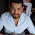# Parallel Reduction in CUDA

Posted by Unknown
Operations which are associative and commutative can be reduction operations some of them are addition, multiplication, bitwise AND/OR/XOR, logical AND/OR/XOR, finding maximum/minimum amongst a given set of numbers. Sequential computation complexity of these operation is over 'n' number of elements is O(n), whereas best theoretical parallel computation complexity can be O(log n). The code below implements parallel reduction in CUDA, here in this case we do addition of floating point array, providing near optimal implementation for arbitrary data sizes and thread block sizes taking transparent scalability into account using shared memory for facilitating faster access and reusing intermediate data.
In case of any questions/clarifications related to this program, please post them in comment, I will be pleased to answer any queries pertaining to it.

#### Parallel Reduction in CUDA

```#include <cuda.h>
#include <stdio.h>
#include <stdlib.h>

#define BLOCK_SIZE 512 // You can change this
#define NUM_OF_ELEMS 4096 // You can change this

#define funcCheck(stmt) {                                            \
cudaError_t err = stmt;                                          \
if (err != cudaSuccess)                                          \
{                                                                \
printf( "Failed to run stmt %d ", __LINE__);                 \
printf( "Got CUDA error ...  %s ", cudaGetErrorString(err)); \
return -1;                                                   \
}                                                                \
}

__global__  void total(float * input, float * output, int len)
{
// Load a segment of the input vector into shared memory
__shared__ float partialSum[2*BLOCK_SIZE];
unsigned int start = 2*blockIdx.x*blockDim.x;

if ((start + t) < len)
{
partialSum[t] = input[start + t];
}
else
{
partialSum[t] = 0.0;
}
if ((start + blockDim.x + t) < len)
{
partialSum[blockDim.x + t] = input[start + blockDim.x + t];
}
else
{
partialSum[blockDim.x + t] = 0.0;
}

// Traverse reduction tree
for (unsigned int stride = blockDim.x; stride > 0; stride /= 2)
{
if (t < stride)
partialSum[t] += partialSum[t + stride];
}

// Write the computed sum of the block to the output vector at correct index
if (t == 0 && (globalThreadId*2) < len)
{
output[blockIdx.x] = partialSum[t];
}
}

int main(int argc, char ** argv)
{
int ii;

float * hostInput; // The input 1D vector
float * hostOutput; // The output vector
float * deviceInput;
float * deviceOutput;

int numInputElements = NUM_OF_ELEMS; // number of elements in the input list
int numOutputElements; // number of elements in the output list
hostInput = (float *) malloc(sizeof(float) * numInputElements);

for (int i=0; i < NUM_OF_ELEMS; i++)
{
}

numOutputElements = numInputElements / (BLOCK_SIZE<<1);
if (numInputElements % (BLOCK_SIZE<<1))
{
numOutputElements++;
}
hostOutput = (float*) malloc(numOutputElements * sizeof(float));

//@@ Allocate GPU memory here
funcCheck(cudaMalloc((void **)&deviceInput, numInputElements * sizeof(float)));
funcCheck(cudaMalloc((void **)&deviceOutput, numOutputElements * sizeof(float)));

// Copy memory to the GPU here
cudaMemcpy(deviceInput, hostInput, numInputElements * sizeof(float), cudaMemcpyHostToDevice);

// Initialize the grid and block dimensions here
dim3 DimGrid( numOutputElements, 1, 1);
dim3 DimBlock(BLOCK_SIZE, 1, 1);

// Launch the GPU Kernel here
total<<<DimGrid, DimBlock>>>(deviceInput, deviceOutput, numInputElements);

// Copy the GPU memory back to the CPU here
cudaMemcpy(hostOutput, deviceOutput, numOutputElements * sizeof(float), cudaMemcpyDeviceToHost);

/********************************************************************
* Reduce output vector on the host
********************************************************************/
for (ii = 1; ii < numOutputElements; ii++)
{
hostOutput += hostOutput[ii];
}

printf("Reduced Sum from GPU = %f\n", hostOutput);

// Free the GPU memory here
cudaFree(deviceInput);
cudaFree(deviceOutput);
free(hostInput);
free(hostOutput);

return 0;
}
```

1.Hi,

Why do you declare start like this "unsigned int start = 2*blockIdx.x*blockDim.x;" ? Won't it push it across the boundary

1.Hi,
I declared start that way so that each threadblock processes unique chunk of numbers from the array as each threadblock is processing "2*blockIdx.x*blockDim.x" numbers for obtaining there reduced sum.
We take care then when "start + anything >= len" those threads don't do any processing so no boundary crossing occurs.

2.3.1.